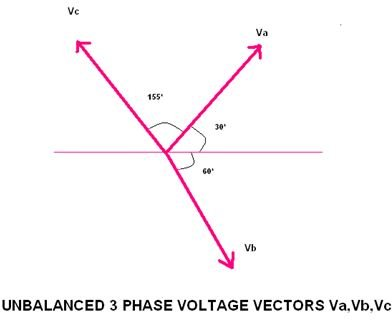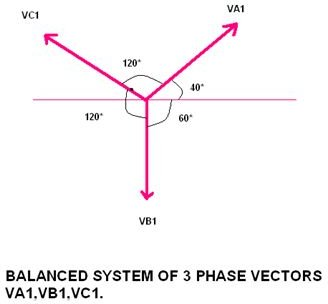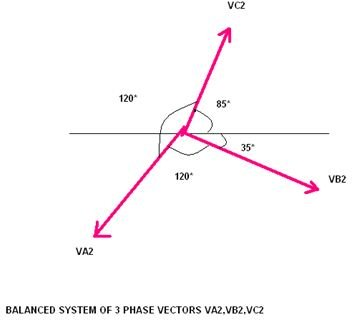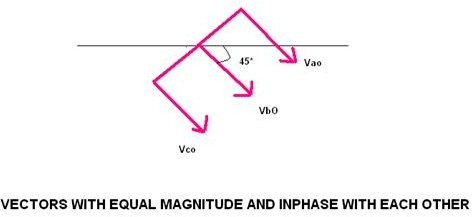# Power in Symmetrical Components

In any 3-phase electric system, if there is an unbalanced vector (whether representing voltages or current), it can be resolved into three balanced systems of vectors which are known as its symmetrical components.

By using this we are able to solve any polyphase (usually 3-phase) circuits having unbalanced vectors for:

• Finding the performance of polyphase electrical machinery when it is operated from a system of unbalanced voltages.
• Calculation of currents resulting from unbalanced faults in the system.

Power flow calculation in symmetrical components solutions is nothing but a voltage and current flow calculation in an unbalanced 3-phase system.

Now let us take an unbalanced 3-phase system which three unbalanced voltage vectors Va, Vb, Vc having phase sequence a-b-c.

The above vectors can be resolved into:

• A balanced system of 3-phase vectors Va1, Vb1, Vc1 having the phase sequence a-b-c, and this is same as that as the original set of three unbalanced vectors, so this is called positive sequence components.
• A balanced system of 3-phase vectors Va2, Vb2, andVc2. The phase sequence of these vectors a-c-b is opposite to that of the original unbalanced vectors, and this is known as negative sequence components.
• A balanced system of three vectors Va3, Vb3, and Vc3. All these vectors are equal in magnitude and are in phase with each other Va3=Vb3 =Vc3s so this is known as zero sequence components.Therefore it has been seen above that an unbalanced 3-phase system of voltages or currents can be regarded as due to two symmetrical 3-phase systems having opposite phase sequence and a system of zero phase sequence.

The resultant vectors, which we get after combining positive sequence vectors and negative sequence vectors, are shown below:

Va = Va1 + Va2 + Va3 ——- (equation 1)

Vb = Vb1 + Vb2 + Vb3——– (equation 2)

Vc = Vc1 + Vc2 + Vc3 ——– (equation 3)

In the above equation, the suffix 1 indicates that the vector belongs to the positive –sequence components and a vector with suffix 2 indicates the vector belongs to the negative- sequence components. Also a vector with suffix 0 indicates that the vector belong to the zero-sequence components.

Usually, these vectors are related to each other with the help of the operator e.

The positive sequence vectors have phase sequence a-b-c and hence they are related as:

Va1 = Va1

Vb1 = e2 Va1

Vc1 = e Va1

The negative sequence vector have phase sequence a-c-b and hence the vectors are related as:

Va2 = Va2

Vc2 = e2 Va2

Vb2 = e Va2

The zero sequence vectors are in phase with each other and have the same magnitude, and hence they are related as:

Va3 = Vb3 =Vc3

Therefore substituting the above in equation 1, 2, and 3 we get

Va = Va1 +Va2 +V3

Vb = e2 Va1 + eVa2 +V3

Vc = e Va1 + e2 Va2 +V3

For our convenience, let us write Va1 as V1, Va2 as V2.

Va =V1+ V2 + V3 ———– (equation4)

Vb = e2V1 + eV2 + V3 ———– (equation5)

Vc = e V1+ e2V2 +V3 ———– (equation6)

An unbalanced 3-phase current can also be similarly linked into its symmetrical component.

Ia =I1 + I2 + I3 ——– (equation7)

Ib = e2 I1 + e I2 + I3———- (equation8)

Ic = e I1+ e2 I2 + I3——— (equation9)

Estimation of Power Flow or Voltage and Current Flow in Symmetrical Components

Estimation of V1 and I1

From equation 8 and equation 9

We can have

e Ib = e3 I1 + e2 I2 + e I3

e2 Ic = e3 I1 + e4 I2 + e2 I3

Now let us assume that e3 =1 and e4 = e

e Ib= I1 + e2 I2 + e I3———- (equation 10)

e2 Ic = I1 + e I2 + e2 I3————(equation 11)

By adding equation 7, equation10, equation11 we get

Ia +e Ib +e2 Ic = I1 +I1 +I2 + I2 + e2 I2 + e I2 + I3 + e I3 +e2 I3

Ia +e Ib +e2 Ic = 2 I1 + I2 ( 1+e+e2) + I3 (1+e+e2)

Ia +e Ib +e2 Ic = 3 I1

I1= 1/3 (Ia +e Ib +e2 Ic)

The same can be applied for V1

V1 = 1/3(Va +e Vb +e2 Vc)

Similarly applying the above methods V2 or I2 and V3 or I3 can be calculated.

V2 = 1/3(Va +e2 Vb + e Vc) and I2 = 1/3(Ia +e2 Ib + e Ic)

V3 = 1/3 (Ia +Ib+Ic) and I3 = 1/3 (Ia +Ib+ Ic)

As we know that power is a product of voltage and current, the power flow can be calculated by using the above relations of vectors and currents.

Power =V1*I1 + V2*I2 +v3*I3

It is clear that the I3 and V3 is one third of the neutral or earth return current or voltage and this will be zero in the unearthed 3-wire electrical system, and also if the vector sum of the current or voltage vectors is zero, then I3 or V3 will be zero.

If there is a flow of positive sequence current components, then there is a flow of negative sequence current, and this is because both are similar, but in case of zero sequence components to flow, then a fourth wire is necessary in the system… so a set of unbalanced 3-phase line to line voltages may be represented by a positive and a negative sequence system of balanced voltages, which are known as symmetrical components.